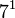# Groups of order 672

(diff) ← Older revision | Latest revision (diff) | Newer revision → (diff)

## Contents

See pages on algebraic structures of order 672| See pages on groups of a particular order

## Statistics at a glace

The number 672 has prime factors 2, 3, and 7. The prime factorization is as follows:$\! 672 = 2^5 \cdot 3^1 \cdot 7^1 = 32 \cdot 3 \cdot 7$

There are both solvable and non-solvable groups of this order. The only possible simple non-abelian composition factor is projective special linear group:PSL(3,2) of order 168, and thus every non-solvable group has composition factors: two copies of cyclic group:Z2 and one copy of$PSL(3,2)$.

Quantity Value Explanation
Total number of groups up to isomorphism 1280
Total number of abelian groups (i.e., finite abelian groups) up to isomorphism 7 (number of abelian groups of order$2^5$)$\times$ (number of abelian groups of order$3^1$)$\times$ (number of abelian groups of order$7^1$) = (number of unordered integer partitions of 5)$\times$ (number of unordered integer partitions of 1)$\times$ (number of unordered integer partitions of 1) =$7 \times 1 \times = 7$.
See classification of finite abelian groups and structure theorem for finitely generated abelian groups.
Total number of nilpotent groups (i.e., finite nilpotent groups) up to isomorphism 51 (number of groups of order 32)$\times$ (number of groups of order 3)$\times$ (number of groups of order 7) =$51 \times 1 \times 1 = 51$.
See number of nilpotent groups equals product of number of groups of order each maximal prime power divisor, which in turn follows from equivalence of definitions of finite nilpotent group.
Number of supersolvable groups up to isomorphism 1134
Total number of solvable groups (i.e., finite solvable groups) up to isomorphism 1272
Number of simple groups up to isomorphism 0
Number of almost simple groups up to isomorphism 0
Number of quasisimple groups up to isomorphism 0
Number of almost quasisimple groups up to isomorphism 2 IDs (672, 1044) and (672, 1045). Both are Schur covering groups for PGL(2,7) and both have SL(2,7) as a normal subgroup of index two.
Number of perfect groups up to isomorphism 0

## GAP implementation

The order 672 is part of GAP's SmallGroup library. Hence, any group of order 672 can be constructed using the SmallGroup function by specifying its group ID. Also, IdGroup is available, so the group ID of any group of this order can be queried.

Further, the collection of all groups of order 672 can be accessed as a list using GAP's AllSmallGroups function.

Here is GAP's summary information about how it stores groups of this order, accessed using GAP's SmallGroupsInformation function:

gap> SmallGroupsInformation(672);

There are 1280 groups of order 672.
They are sorted by their Frattini factors.
1 has Frattini factor [ 42, 1 ].
2 has Frattini factor [ 42, 2 ].
3 has Frattini factor [ 42, 3 ].
4 has Frattini factor [ 42, 4 ].
5 has Frattini factor [ 42, 5 ].
6 has Frattini factor [ 42, 6 ].
7 - 47 have Frattini factor [ 84, 7 ].
48 - 114 have Frattini factor [ 84, 8 ].
115 - 133 have Frattini factor [ 84, 9 ].
134 has Frattini factor [ 84, 10 ].
135 has Frattini factor [ 84, 11 ].
136 - 176 have Frattini factor [ 84, 12 ].
177 - 217 have Frattini factor [ 84, 13 ].
218 - 258 have Frattini factor [ 84, 14 ].
259 - 277 have Frattini factor [ 84, 15 ].
278 - 281 have Frattini factor [ 168, 45 ].
282 - 285 have Frattini factor [ 168, 46 ].
286 - 371 have Frattini factor [ 168, 47 ].
372 - 378 have Frattini factor [ 168, 48 ].
379 - 386 have Frattini factor [ 168, 49 ].
387 - 721 have Frattini factor [ 168, 50 ].
722 - 745 have Frattini factor [ 168, 51 ].
746 - 752 have Frattini factor [ 168, 52 ].
753 - 760 have Frattini factor [ 168, 53 ].
761 - 846 have Frattini factor [ 168, 54 ].
847 - 932 have Frattini factor [ 168, 55 ].
933 - 1018 have Frattini factor [ 168, 56 ].
1019 - 1042 have Frattini factor [ 168, 57 ].
1043 - 1045 have Frattini factor [ 336, 208 ].
1046 - 1048 have Frattini factor [ 336, 209 ].
1049 has Frattini factor [ 336, 210 ].
1050 has Frattini factor [ 336, 211 ].
1051 - 1065 have Frattini factor [ 336, 212 ].
1066 has Frattini factor [ 336, 213 ].
1067 - 1077 have Frattini factor [ 336, 214 ].
1078 - 1088 have Frattini factor [ 336, 215 ].
1089 - 1103 have Frattini factor [ 336, 216 ].
1104 - 1114 have Frattini factor [ 336, 217 ].
1115 - 1125 have Frattini factor [ 336, 218 ].
1126 - 1178 have Frattini factor [ 336, 219 ].
1179 - 1184 have Frattini factor [ 336, 220 ].
1185 - 1191 have Frattini factor [ 336, 221 ].
1192 - 1198 have Frattini factor [ 336, 222 ].
1199 - 1200 have Frattini factor [ 336, 223 ].
1201 - 1202 have Frattini factor [ 336, 224 ].
1203 - 1217 have Frattini factor [ 336, 225 ].
1218 - 1232 have Frattini factor [ 336, 226 ].
1233 - 1247 have Frattini factor [ 336, 227 ].
1248 - 1253 have Frattini factor [ 336, 228 ].
1254 - 1280 have trivial Frattini subgroup.

For the selection functions the values of the following attributes
are precomputed and stored:
IsAbelian, IsNilpotentGroup, IsSupersolvableGroup, IsSolvableGroup,
LGLength, FrattinifactorSize and FrattinifactorId.

This size belongs to layer 2 of the SmallGroups library.
IdSmallGroup is available for this size.